### Home > PC > Chapter 8 > Lesson 8.2.3 > Problem8-113

8-113.
1. Solve each equation for the exact value of x. Homework Help ✎1. ln x = 3

2. ex = 200

3. 5 ln(x + 1) = 20

4. e(x+2) + 20 = 100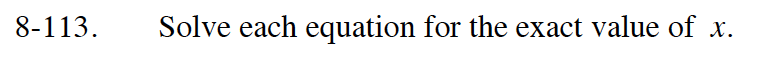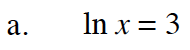elnx = e3

x = e3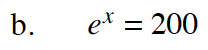lnex = ln200

x = ln200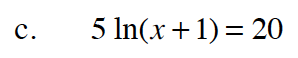ln(x+1) = 4
eln(x+1) = e4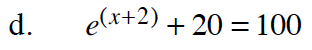e(x+2) = 80
lne(x+2) = ln80